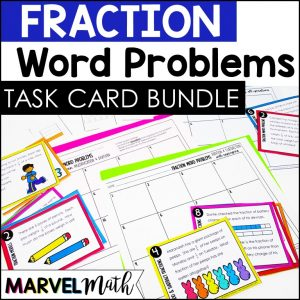Save 10% on your first order with code EXTRA10

# Multiply & Divide Whole Numbers and Unit Fractions Task Cards

\$2.00\$5.00
SKU: 3035181 Categories: ,

## Description

Your students will get engaging practice Multiplying and Dividing Fractions by Whole Numbers with this resource. Students will solve word problems involving multiplying fractions by whole numbers or dividing fractions by whole numbers and find the matching model or number line for each problem. The models are based on the types of models used in released STAAR tests.

Save money on this resource by purchasing it in the 5th Grade Fraction Word Problem Bundle!

What’s Included?

• 24 Model Cards
• Color and BW versions
• Teacher Key
• Student Recording Sheets
• Task Card sized cover and key for easy organization

Teachers Like You Said

?????????? Great questions! Definitely helped review before state testing in fun way. Thank you for this great resource! 🙂 -Angel C.

?????????? LOVED this! Great practice for the STAAR. – Dana H.

?????????? My students were really struggling with this concept and the visuals were so helpful! Thank you! -Jamie H.

?????????? My students enjoyed matching the models to the real world problems. Thank you for creating an engaging activity that all learners can use. -Elizabeth R.

You may also be interested in this very popular No Prep resource:

 Guided Math Lessons and Stations for Dividing Fractions

Standards Alignment:

TEKS:

5.3I Represent and solve multiplication of a whole number and a fraction that refers to the same whole using objects and pictorial models, including area models.

5.3J Represent division of a unit fraction by a whole number and the division of a whole number by a unit fraction such as 1/3 ÷ 7 and 7 ÷ 1/3 using objects and pictorial models, including area models.

5.3L Divide whole numbers by unit fractions and unit fractions by whole numbers.

Common Core:

5.NF.B.6 Solve real world problems involving multiplication of fractions and mixed numbers, e.g., by using visual fraction models or equations to represent the problem.

5.NF.B.7.A Interpret division of a unit fraction by a non-zero whole number, and compute such quotients. For example, create a story context for (1/3) ÷ 4, and use a visual fraction model to show the quotient. Use the relationship between multiplication and division to explain that (1/3) ÷ 4 = 1/12 because (1/12) × 4 = 1/3

5.NF.B.7.B Interpret division of a whole number by a unit fraction, and compute such quotients. For example, create a story context for 4 ÷ (1/5), and use a visual fraction model to show the quotient. Use the relationship between multiplication and division to explain that 4 ÷ (1/5) = 20 because 20 × (1/5) = 4.